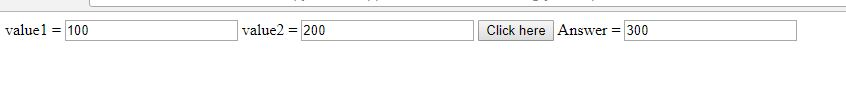# Addition of two numbers using JavaScript

In this JavaScript program, we are going to learn how to take input from HTML form and print add (sum) of given numbers calculating through JavaScript?
Submitted by Ridhima Agarwal, on October 18, 2017

Here, we are taking input from HTML form (using input tags) and printing the sum (addition) on the HTML by calculating it through JavaScript.

The JavaScript code will be called on button submit event, when we will click on the button (which is placed on the HTML file).

Consider this HTML code

```value1 = <input type="text" id="value1" name="value1" value=""/>
value2 = <input type="text" id="value2" name="value2" value=""/>
```

There are two ids value1 and value2 which are going to be used in the JavaScript, JavaScript function will get the values from these input tags by using given ids (value1 and value2).

JavaScript code to add these values

```<script language="javascript">
{
var val1 = parseInt(document.getElementById("value1").value);
var val2 = parseInt(document.getElementById("value2").value);
ansD.value = val1 + val2;
}
</script>
```

Note: JavaScript code is written within in the <script> ... </script> tag.

Here, we are defining a function addNumbers() which is getting the value of first input using parseInt(document.getElementById("value1").value); and second input using parseInt(document.getElementById("value2").value); values are getting stored in variables val1 and val2.

Variable ansD is getting initialized with the element having id answer, which is the id of third input box where we are going to be display the result.

Finally, we are assigning the sum (addition) of val1 and val2, which will display within the third input box.

See DEMO here,

DEMO

HTML code with the JavaScript

```<html>
<script language="javascript">
{
var val1 = parseInt(document.getElementById("value1").value);
var val2 = parseInt(document.getElementById("value2").value);
ansD.value = val1 + val2;
}
</script>
<body>
value1 = <input type="text" id="value1" name="value1" value=""/>
value2 = <input type="text" id="value2" name="value2" value=""/>
</body>
</html>
```

Output (Result)